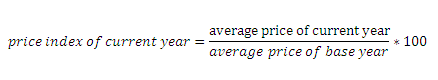# Price index number

Price index number is the relative price level of current year to price level of base year. The price index is constructed in order to know the relative price levels of different years. It gives the change in value of money in percentage. To construct price index we rake the price level of one of the past year as base price level. The year is called base year. The price level of base year is indexed as 100. Then the price index of the current year is calculated using the formula:Average price is known a general price level. It is average prices of goods and services transacted in the base year and current year. To calculate average price the commodities may be given equal or more and less weights. The price index is constructed giving equal weights to all commodities is called simple price index. The price index constructed by giving different weights to different commodities is called weighted price index.